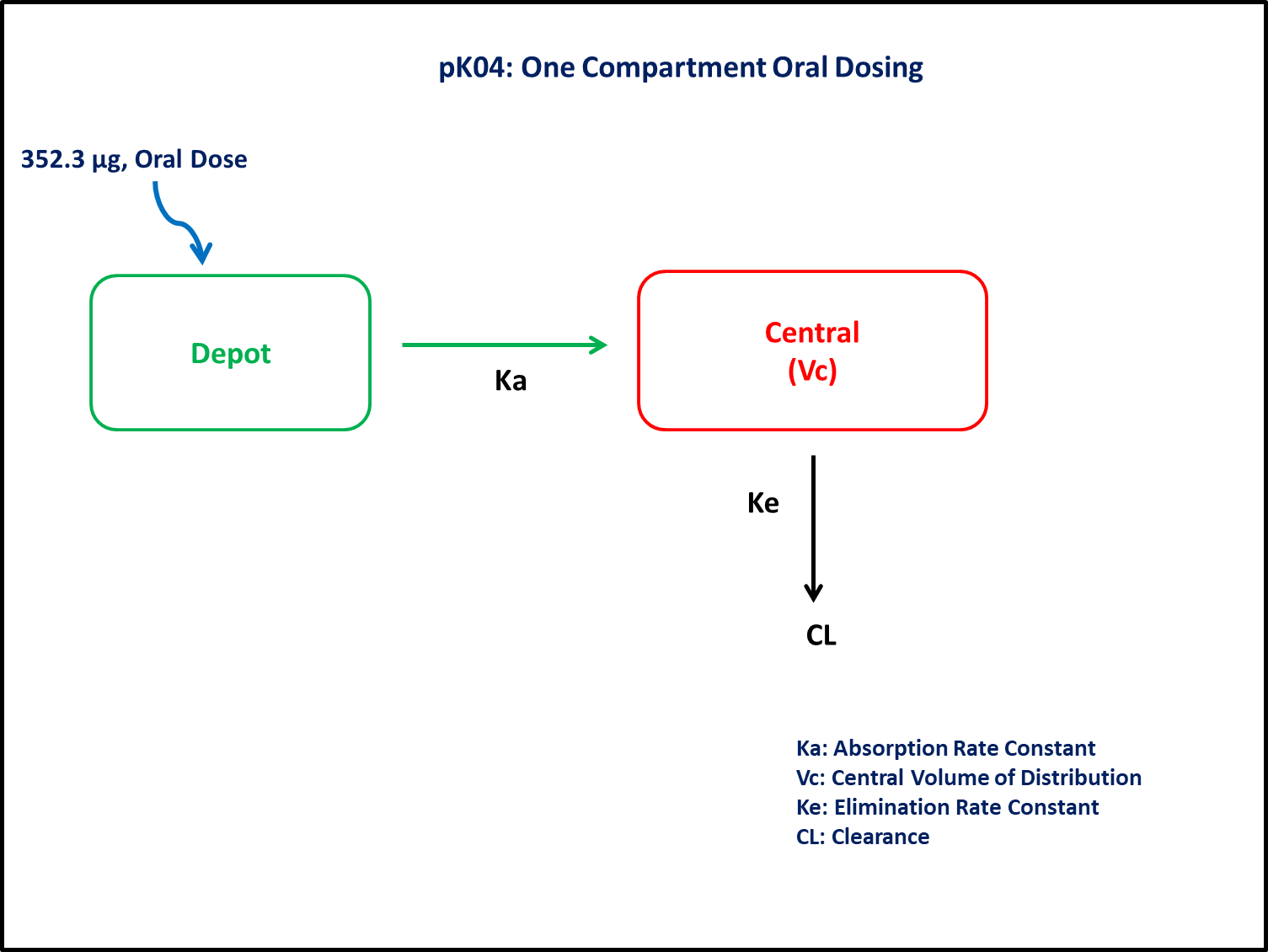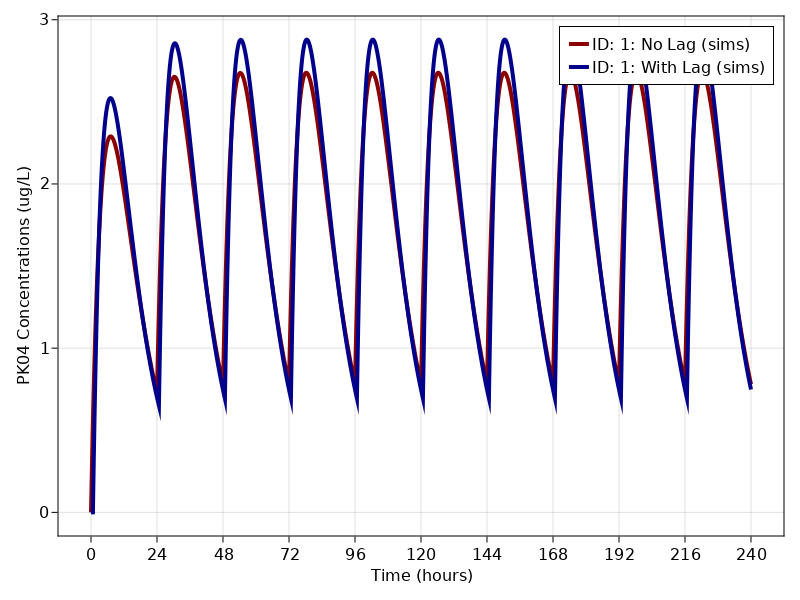# Exercise PK04 - One compartment oral dosing

### Background

• Structural model - One compartment linear elimination with first order absorption.

• Route of administration - Oral, Multiple dosing

• Dosage Regimen - 352.3 μg

• Number of Subjects - 1### Learning Outcome

This is a one compartment multiple oral dosing model. In the exercise pk04, four models are compared.

• Model 1 - One compartment model without lag-time, distinct parameters Ka and K

• Model 2 - One compartment model with lag time, distinct parameters Ka and K

• Model 3 - One compartment model without lag time, Ka = K = K¹

• Model 4 - One compartment model with lag time, Ka = K = K¹

### Objectives

In this tutorial, you will learn how to build one compartment model for multiple oral dosing and to simulate the model for a single subject.

### Libraries

call the "necessary" libraries to get start.

using Pumas
using PumasUtilities
using Random
using CairoMakie


### Model 1 & 2

In this one compartment model, we administer multiple doses orally.

pk_04_1_2    = @model begin
desc = "One Compartment Model"
timeu = u"hr"
end

@param begin
"Absorption Rate constant (1/hr)"
tvka     ∈ RealDomain(lower=0)
"Elimination Rate Constant (1/hr)"
tvk      ∈ RealDomain(lower=0)
"Volume of Distribution (L)"
tvvc     ∈ RealDomain(lower=0)
"Lag-time (hr)"
tvlag    ∈ RealDomain(lower=0)
Ω        ∈ PDiagDomain(3)
"Proportional RUV"
σ²_prop  ∈ RealDomain(lower=0)
end

@random begin
η        ~ MvNormal(Ω)
end

@pre begin
Ka       = tvka * exp(η)
K        = tvk * exp(η)
Vc       = tvvc * exp(η)
end

@dosecontrol begin
lags     = (Depot = tvlag,)
end

@dynamics begin
Depot'   = -Ka*Depot
Central' =  Ka*Depot - K*Central
end

@derived begin
"""
PK04 Concentration (ug/L)
"""
cp       = @. Central/Vc
"""
PK04 Concentration (ug/L)
"""
dv       ~ @. Normal(cp, sqrt(cp^2*σ²_prop))
end
end

PumasModel
Parameters: tvka, tvk, tvvc, tvlag, Ω, σ²_prop
Random effects: η
Covariates:
Dynamical variables: Depot, Central
Derived: cp, dv
Observed: cp, dv


### Parameters

The parameters are as given below. tv represents the typical value for parameters.

• $Ka$ - Absorption Rate Constant (hr⁻¹),

• $K$ - Elimination Rate Constant (hr⁻¹),

• $Vc$ - Volume of Central Compartment(L),

• $Ω$ - Between Subject Variability,

• $σ$ - Residual error

param = [
(tvka    = 0.14,
tvk     = 0.14,
tvvc    = 56.6,
tvlag   = 0,
Ω       = Diagonal([0.0,0.0,0.0]),
σ²_prop = 0.015),

(tvka    = 0.20,
tvk     = 0.12,
tvvc    = 64.9,
tvlag   = 0.70,
Ω       = Diagonal([0.0,0.0,0.0]),
σ²_prop = 0.01)
]

2-element Vector{NamedTuple{(:tvka, :tvk, :tvvc, :tvlag, :Ω, :σ²_prop), T}
where T<:Tuple}:
(tvka = 0.14, tvk = 0.14, tvvc = 56.6, tvlag = 0, Ω = [0.0 0.0 0.0; 0.0 0.
0 0.0; 0.0 0.0 0.0], σ²_prop = 0.015)
(tvka = 0.2, tvk = 0.12, tvvc = 64.9, tvlag = 0.7, Ω = [0.0 0.0 0.0; 0.0 0
.0 0.0; 0.0 0.0 0.0], σ²_prop = 0.01)


### Dosage Regimen

Subject received 352.3 μg of oral dose once a day for 10 days.

ev1  = DosageRegimen(352.3, time = 0, ii = 24, addl = 9, cmt = 1)
pop  = map(i -> Subject(id = i, events = ev1, observations = (cp = nothing,)), ["1: No Lag", "1: With Lag"])


### Simulation

Simulation the plasma concentration of the drug after multiple oral dosing

Random.seed!(123)
sim = map(zip(pop, param)) do (subj, p)
return simobs(pk_04_1_2, subj, p, obstimes = 0:0.1:240)
end


### Visualize results

f, a, p = sim_plot(pk_04_1_2, sim,
observations=:cp,
linewidth = 4,
color = :redsblues,
axis = (xlabel = "Time (hours)",
ylabel = "PK04 Concentrations (ug/L)",
xticks = 0:24:240,))
axislegend(a)
f